# CSE 130 SP16- Homework #2 (140 pts)

## Due by 23:59 pm on Friday, April 22th, 2016.

NOTE: To get the code provided for problem 2 to work on Windows/Mac please install ImageMagick Remember that this is only to enable you to play with the assignment at home.

## Overview

The objective of this assignment is for you to have fun learning about recursion, recursive datatypes, and make some pretty cool pictures.All the problems require relatively little code ranging from 2 to 10 lines. If any function requires more than that, you can be sure that you need to rethink your solution.

The assignment is in the file hw2.ml that you need to download, edit and submit. As before, your task is to replace each expression of the form

``failwith "to be written"``

with the the appropriate OCaml code for each of those expressions.

Note: All the solutions can be done using the purely functional fragment of OCaml, using constructs covered in class, and most require the use of recursion. Solutions using imperative features such as references, while loops or library functions will receive no credit It is a good idea to start this assignment early; ML programming, while quite simple (when you know how), often seems somewhat foreign at first, particularly when it comes to recursion and list manipulation.

## Assignment Testing and Evaluation

Your functions/programs must compile and run with `ocaml-top` on `ieng6.ucsd.edu`.

Most of the points, will be awarded automatically, by evaluating your functions against a given test suite. hw2.ml contains a very small suite of tests which gives you a flavor of of these tests.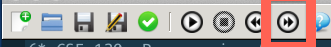At any point, hit fast-forward button (shown above) labeled run the current program as far as possible to get a report on how your code stacks up against the simple tests.

The last line of the interactive shell must contain the word:

``````130>>Compiled

- : int * int = (SCORE, TOTAL) ``````

where `SCORE` and `TOTAL` are a pair of integers, reflecting your score and the max possible score on the sample tests.

If for some problem, you cannot get the code to compile, leave it as is with the `failwith ...` with your partial solution enclosed below as a comment.

The other lines will give you a readout for each test. You are encouraged to try to understand the testing code, but you will not be graded on this.

## Submission Instructions

1. Keep your `hw2.ml` loaded (and saved) in the editor pane,

2. Hit the green tick mark button to submit your code.`turnin` will provide you with a confirmation of the submission process; make sure that the size of the file indicated by `turnin` matches the size of your file. See the ACS Web page on turnin for more information on the operation of the program.

## Problem #1: Tail Recursion

We say that a function is tail recursive if every recursive call is a tail call whose value is immediately returned by the procedure. See these two handouts for more details on what a tail-recursive function is in Ocaml.

### (a) 15 points

Without using any built-in ML functions, write a tail-recursive Ocaml function

``val assoc : int * string * (string * int) list -> int ``

or more generally,

``val assoc : 'a * 'b * ('b * 'a) list -> 'a ``

that takes a triple `(v, k, [(k1,v1);...;(kn,vn)])` and finds the first `ki` that equals `k`. If such a `ki` is found, then the function should return `vi`, otherwise, the default value `v` is returned.

Once you have implemented the function, you should get the following behavior at the ML prompt:

``````# assoc (-1,"william",[("ranjit",85);("william",23);("moose",44)]);;
- : int = 23

# assoc (-1,"bob",[("ranjit",85);("william",23);("moose",44)]);;
- : int = -1 ``````

### (b) 15 points

Without using any built-in ML functions, modify the skeleton for `removeDuplicates` to obtain a function of type

``val removeDuplicates : int list -> int list``

or more generally,

``val removeDuplicates : 'a list -> 'a list``

such that `removeDuplicates xs` returns the list of elements of `xs` with the duplicates, i.e. second, third, etc. occurrences, removed, and where the remaining elements appear in the same order as in `xs`.

For this function only, you may use the library functions `List.rev` and `List.mem`. Once you have implemented the function, you should get the following behavior at the ML prompt:

``````# removeDuplicates [1;6;2;4;12;2;13;6;9];;
- : int list = [1;6;2;4;12;13;9]``````

### (c) 20 points

Without using any built-in ML functions, or the `while` or `for` construct, write a tail-recursive ML function:

``val wwhile : (int -> int * bool) * int -> int``

or more generally,

``val wwhile : ('a -> 'a * bool) * 'a -> 'a ``

such that `wwhile (f, x)` returns `x'` where there exist values `v_0`,…,`v_n` such that

• `x` is equal to `v_0`
• `x'` is equal to `v_n`
• for each `i` between `0` and `n-2`, we have `f v_i` equals `(v_i+1, true)`
• `f v_n-1` equals `(v_n, false)`.

Your function should be tail recursive. Once you have implemented the function, you should get the following behavior at the ML prompt:

``````# let f x = let xx = x*x*x in (xx, xx < 100) in wwhile (f,2);;
- : int = 512``````

### (d) 20 points

Without using any built-in ML functions, modify the skeleton for `fixpoint` to obtain a function

``val fixpoint: (int -> int) * int -> int ``

or more generally,

``val fixpoint: ('a -> 'a) * 'a -> 'a ``

such that `fixpoint (f, x0)` returns the first `xi` where

• `xi` is equal tyo `f x_i-1`, and, furthermore,
• `f xi` is equal to `xi`

Once you have implemented the function, you should get the following behavior at the ML prompt:

``````# let g x = truncate (1e6 *. cos (1e-6 *. float x));;
val f : int -> int = fn

# fixpoint (g, 0);;
- : int = 739085

# let collatz n = match n with 1 -> 1 | _ when n mod 2 = 0 -> n/2 | _ -> 3*n + 1;;
val collatz: int -> int = fn

# fixpoint (collatz, 1) ;;
- : int = 1
# fixpoint (collatz, 3) ;;
- : int = 1
# fixpoint (collatz, 48) ;;
- : int = 1
# fixpoint (collatz, 107) ;;
- : int = 1
# fixpoint (collatz, 9001) ;;
- : int = 1``````

## Problem #2: Random Art

At the end of this assignment, you should be able to produce pictures of the kind shown below. To do so, we shall devise a grammar for a certain class of expressions, design an ML datatype whose values correspond to such expressions, write code to evaluate the expressions, and then write a function that randomly generates such expressions and plots them thus producing random psychedelic art.

Color Images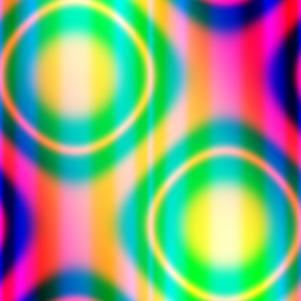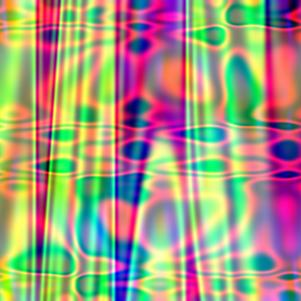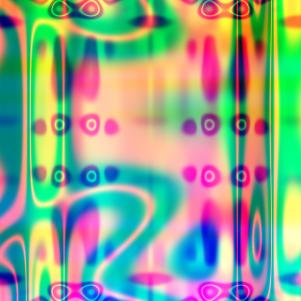Gray Scale Images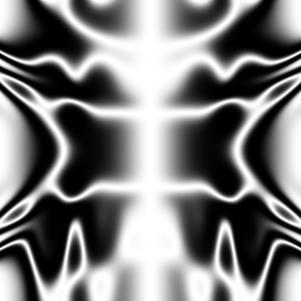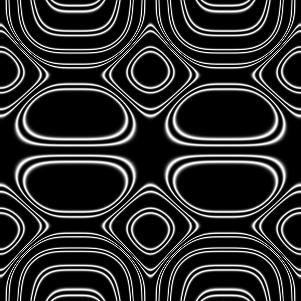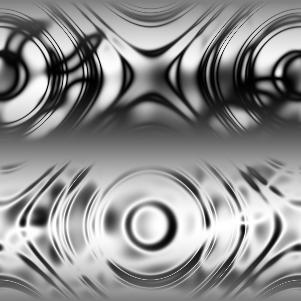### (a) 15 points

The expressions described by the grammar:

``````e ::= x
| y
| sin (pi*e)
| cos (pi*e)
| ((e + e)/2)
| e * e
| (e<e ? e : e)``````

where pi stands for the constant 3.142, are functions over the variables x,y, which are guaranteed to produce a value in the range [-1,1] when x and y are in that range. We can represent expressions of this grammar in ML using values of the following datatype:

``````type expr = VarX
| VarY
| Sine of expr
| Cosine of expr
| Average of expr * expr
| Times of expr * expr
| Thresh of expr * expr * expr * expr ``````

First, write a function

``val exprToString : expr -> string``

to enable the printing of expressions. Once you have implemented the function, you should get the following behavior at the ML prompt:

``````# let sampleExpr1 = Thresh(VarX,VarY,VarX,(Times(Sine(VarX),Cosine(Average(VarX,VarY)))));;
- : expr =  ...

# exprToString sampleExpr1
- : string = "(x<y?x:sin(pi*x)*cos(pi*((x+y)/2)))"``````

### (b) 15 points

Next, write a function

``val eval : expr * float * float -> float``

such that `eval (e, vx, vy)` returns the result of evaluating the expression `e` at the point `(vx, vy)` that is, evaluating the result of `e` when `VarX` has the value `vx` and `VarY` has the value `vy`. You should use Ocaml functions like, `sin`, and `cos` to build your evaluator. Recall that `Sine(VarX)` corresponds to the expression `sin(pi*x)`

Once you have implemented the function, you should get the following behavior at the ML prompt:

``````# eval (Sine(Average(VarX,VarY)),0.5,-0.5);;
- : float = 0.0

# eval (Sine(Average(VarX,VarY)),0.3,0.3);;
- : float = 0.809016994375

# eval (sampleExpr,0.5,0.2);;
- : float = 0.118612572815``````

Uncomment and execute the line:

``let _ = emitGrayscale (eval_fn sampleExpr, 150, "sample") ;;``

to generate the grayscale image `art_g_sample.jpg` in your working directory. To receive full credit, this image must look like the leftmost grayscale image displayed above. Note that this requires your implementation `eval` to work correctly. A message `Uncaught exception ...` is an indication that your `eval` is returning a value outside the valid range `[-1.0,1.0]`.

### (c) 20 points

Next, you must fill in an implementation for the function

``val build: ((int * int -> int) * int) -> expr``

The function `build` is called with the pair of arguments `(rand, depth)`.

• `rand` is a random number generator of type `int * int -> int`. Each call `rand (i,j)` returns a random integer between `i` and `j-1` inclusive. Use this function to randomly select operators when composing subexpressions to build up larger expressions.

• `depth` is a a maximum nesting dept; a random expression of depth `d` is built by randomly composing sub-expressions of depth `d-1` and the only expressions of depth `0` are `VarX` and `VarY`.

With this in place you can generate random art using the functions

``````val doRandomGray : int * int * int -> unit
val doRandomColor : int * int * int -> unit``````

Each function takes as a parameter a triple `(depth, seed1, seed2)` where `depth` is the depth of the expression to be generated and `seed1`, `seed2` are two seeds for the random number generator. The functions generate JPEG files called `art_g_<depth>_<seed1>_<seed2>.jpg` and `art_c_<depth>_<seed1>_<seed2>.jpg` respectively. The first is a gray scale image, built by mapping out a single randomly generated expression over the plane, and the second is a color image built using three functions for the intensities of red, green and blue.

Play around with how you generate the expressions, using the tips below.

• Depths of 8-12 produce interesting pictures, but play around!
• Make sure your expressions don’t get cut-off early with `VarX`, `VarY` as small expressions give simple pictures.
• Play around to bias the generation towards more interesting operators.

Save the parameters (i.e. the depth and the seeds) for your best three color images in the bodies of `c1`, `c2`, `c3` respectively, and best three gray images in `g1`, `g2` , `g3`.

### (d) 20 points

Finally, add two new operators to the grammar, i.e. to the datatype, by introducing two new datatype constructors, and adding the corresponding cases to `exprToString`, `eval`, and `build`. The only requirements are that the operators must return values in the range `[-1.0,1.0]` if their arguments (ie `VarX` and `VarY`) are in that range, and that one of the operators take three arguments, i.e. one of the datatype constructors is of the form: `C of expr * expr * expr` You can include images generated with these new operators when choosing your best images for part (c).

### (e) Extra Credit: 15 points

The creators of the best five images, will get extra credit. Be creative!